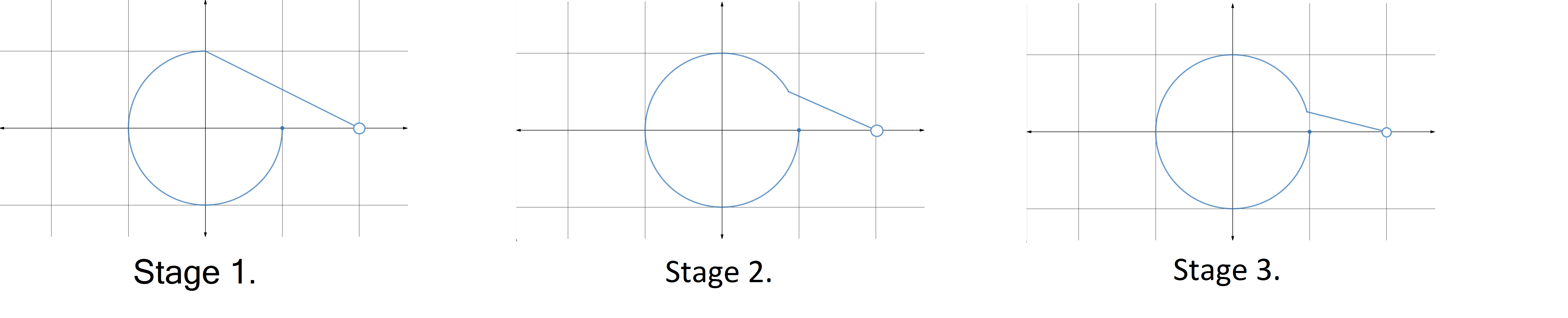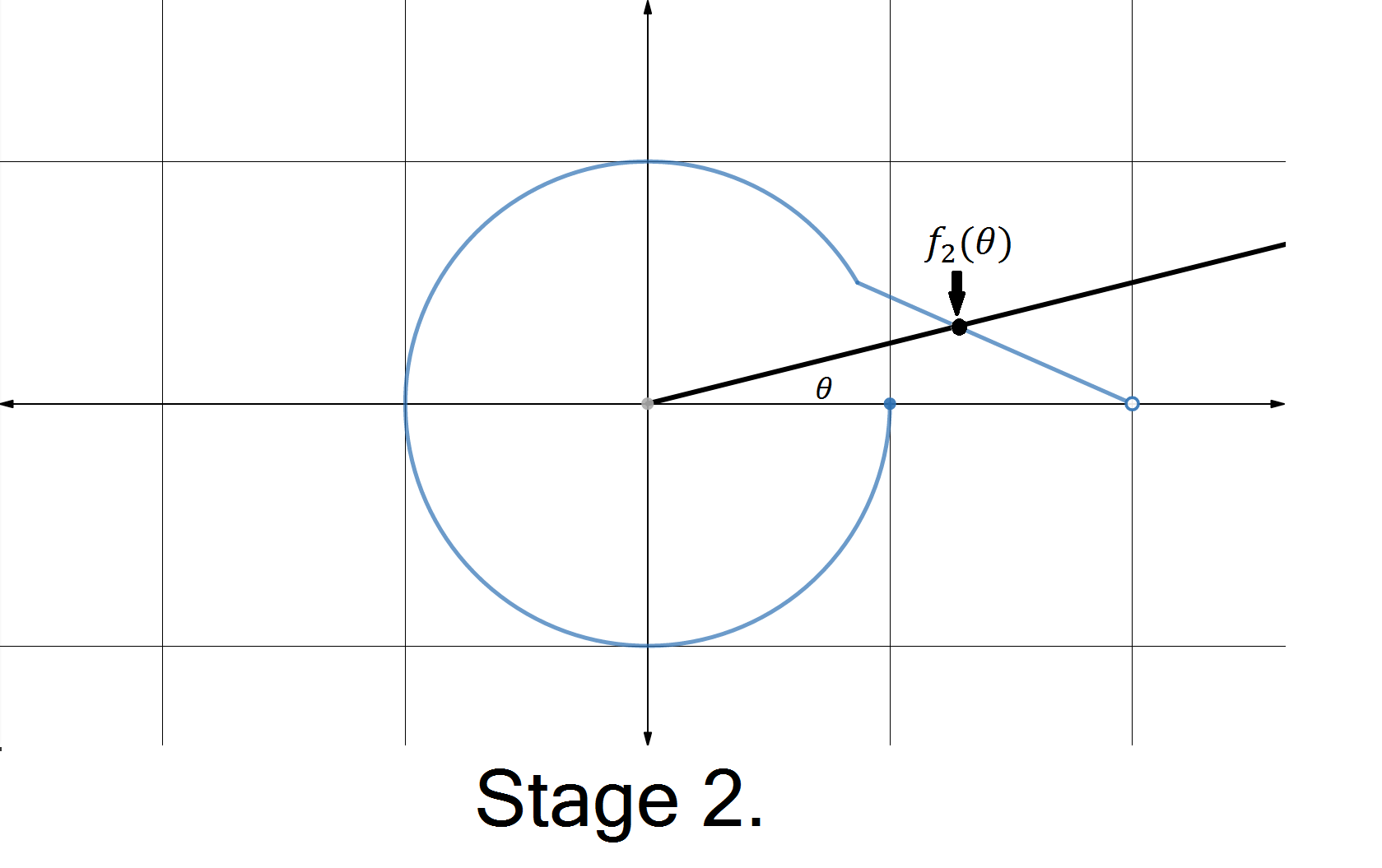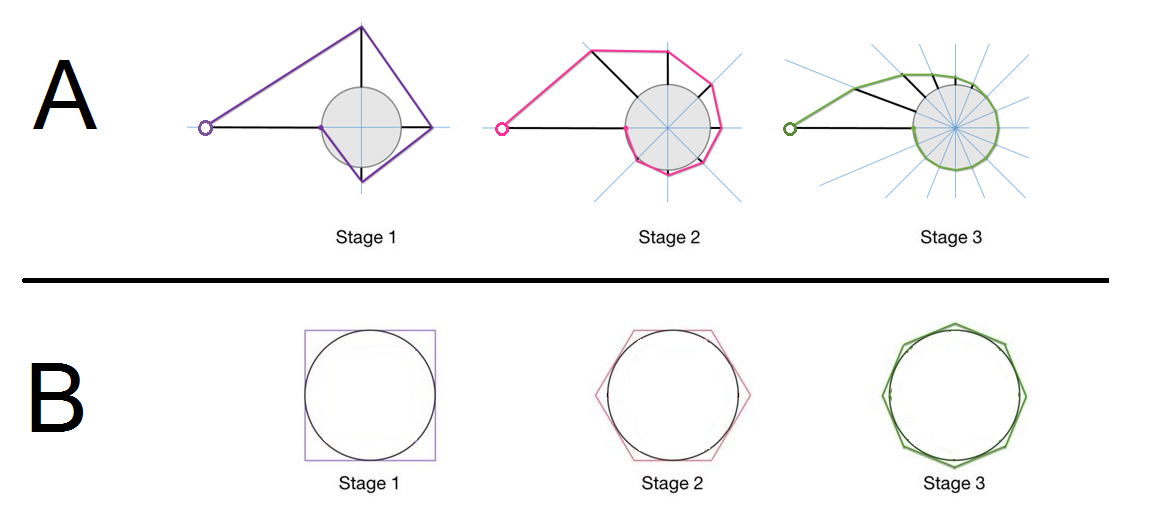Geometry

# Deep Dive: Pointwise and Uniform Convergence 2A sequence of curves that approximate the unit circle is shown.

True or False? These curves converge pointwise to the unit circle.

Details.

There is an open circle at the point $(2,0),$ and a linear portion with slope $\frac{-1}{n+1}$ at Stage $n$ that continues until it meets the unit circle, and then follows the circle around counterclockwise to $(1,0).$

Let $f_n(\theta)$ be the point where a ray making an angle of $\theta$ with the positive $x-$axis intersects the Stage $n$ curve $f_n,$ and let $f(\theta)$ be the point where the same ray intersects the unit circle. A picture of $f_2(\theta)$ for an angle $\theta$ is shown below.

True or False? For each $\theta$ in the interval $[0, 2\pi),$

$\lim_{n \to \infty} f_n(\theta) = f(\theta).$A sequence of curves that approximate the unit circle is shown.

True or False? The derivatives of the curves converge to the derivative of the circle.

Details. There is an open circle at the point $(2,0),$ and a linear portion with slope $\frac{-1}{n+1}$ at Stage $n$ that continues until it meets the unit circle, and then follows the circle around counterclockwise to $(1,0).$

Let $d_n(\theta)$ be the derivative $\frac{dy}{dx}$ of the Stage $n$ curve at the point where it intersects a ray making an angle of $\theta$ with the positive $x-$axis, and let $d(\theta)$ be the derivative $\frac{dy}{dx}$ of the unit circle at the point where the same ray intersects the unit circle.

True or False? For each $\theta$ in the interval $(0, 2\pi),$

$\lim_{n \to \infty} d_n(\theta) = d(\theta).$A sequence of curves that approximate the unit circle is shown (as the stages progress, the slope of the linear portion approaches 0.)

True or False? Let $L(f_n)$ represent the length of the Stage $n$ curve, and $L(f)$ represent the length of the unit circle. Then

$\lim_{n \to \infty} L(f_n) = L(f).$A sequence of curves that approximate the unit circle is shown (as the stages progress, the slope of the linear portion approaches 0.)

True or False? The derivatives of the curves converge uniformly to the derivative of the circle.

Details. Let $d_n(\theta)$ be the derivative $\frac{dy}{dx}$ of the Stage $n$ curve at the point where it intersects a ray making an angle of $\theta$ with the positive $x-$axis, and let $d(\theta)$ be the derivative $\frac{dy}{dx}$ of the unit circle at the point where the same ray intersects the unit circle.

True or False? For each $\theta$ in the interval $(0, 2\pi),$ the sequence $\{d_n(\theta)\}$ converges uniformly to $d(\theta).$

Note. Uniform convergence in this example is equivalent to:

For every $\epsilon > 0,$ there is a natural number $N_{\epsilon}$ such that

$n>N_{\epsilon} \Rightarrow \left|d_n(\theta) - d(\theta) \right| < \epsilon \text{ for all } \theta \text{ in } (0, 2\pi).$Two sequences of curves are shown. In both cases, we have a sequence of curves that is converging pointwise to the unit circle, and whose derivatives are converging pointwise to the corresponding derivative of the unit circle.

In which case is the convergence uniform and the lengths of the curves converge to the length of the unit circle?

×